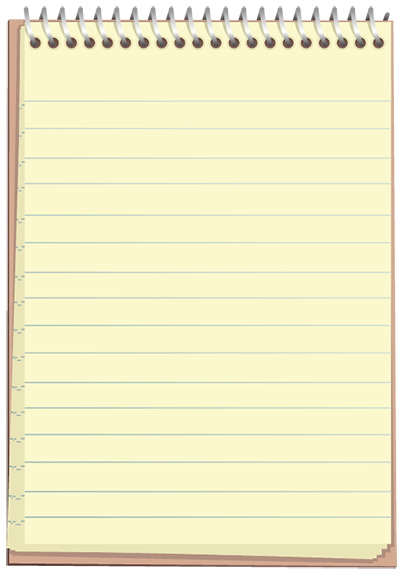Forces and Motion

Equation

acceleration = velocity      time

a =  v t

1.3

Equation

velocity =

acceleration x time

v = at

1.4

Equation

final velocity = initial velocity + acceleration x time

vf = vi + at

1.5

Acceleration

NotebookNotes• Acceleration is the change in velocity over time. • Because it is time dependent, all acceleration is an average. • Applying an unbalanced force to an object induces an acceleration.

Equation

average velocity = initial velocity + final velocity ÷ two

vave = (vi + vf) / 2

1.6

Equation

distance traveled  =  ½ x acceleration x times squared

d = ½ at2

1.7